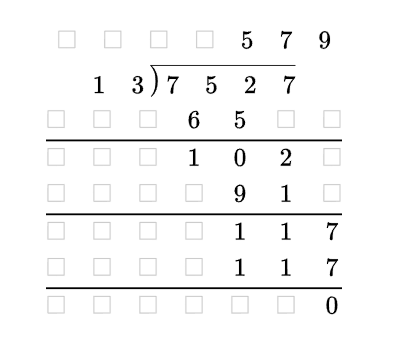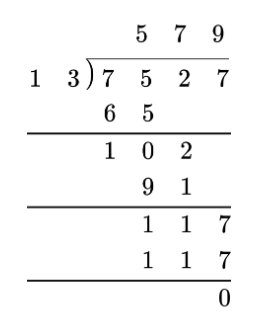# Showing work for a long division problem?

I can create a problem with the long division symbol, but can’t create additional lines to show the steps. Is there way to create a long division problem and be able to show the work? I use a matrix to do this for addition, subtraction and multiplication but am struggling with using a matrix for long division.

Hi,

we do not have a particular form for creating long divisions. However, it can be composed of 3 objects put together into centered multiline environment. It’s a bit tricky, because alignment looks different in the editor and in the final math.

Here is an example:

• in editor:• inserted math:Steps:

• press Enter and choose Centered
• in the first row insert a matrix (without brackets) with 1 row and n+1 columns (+1 for better alignment)
• in the second row insert long division command and on then insert a 1xk matrix on the left and 1xm matrix on the right (where k+m = n). Add 2 spaces on each side offor better alignment
• press Enter to create the third row and insert lx(n+1) matrix, add vertical line for every second row using the matrix menu on the left

Mathcode:

\begin{gather*}
{\begin{matrix}
{}&{}&{}&{}&{5}&{7}&{9}\\
\end{matrix}}\\
{\ldiv{\begin{matrix}
{1}&{3\,}\\
\end{matrix}}{\begin{matrix}
{\,7}&{5}&{2}&{7}\\
\end{matrix}}}\\
{\begin{matrix}
{}&{}&{}&{6}&{5}&{}&{}\\
\hline
{}&{}&{}&{1}&{0}&{2}&{}\\
{}&{}&{}&{}&{9}&{1}&{}\\
\hline
{}&{}&{}&{}&{1}&{1}&{7}\\
{}&{}&{}&{}&{1}&{1}&{7}\\
\hline
{}&{}&{}&{}&{}&{}&{0}\\
\end{matrix}}
\end{gather*}


(to insert this math into editor, copy it the whole mathcode above, then paste it in the empty editor)